# Abstracts

Growth functions with upper horizontal asymptote do not have a maximum point, but we frequently question from which point growth can be considered practically constant, that is, from which point the curve is sufficiently close to its asymptote, so that the difference can be considered non-significant. Several methods have been employed for this purpose, such as one that verifies the significance of the difference between the curve and its asymptote using a t-test, and that of Portz et al. (2000), who used segmented regression. In the present work, we used logistic growth function, which has horizontal asymptote and one inflection point, and applied a new method consisting in the mathematical determination of a point in the curve from which the growth acceleration asymptotically tends to zero. This method showed the advantage to have biological meaning besides leading to a point quite close to those obtained using the beforementioned methods.

nonlinear regression; logistic model; critical point of growth

Em funções de crescimento que apresentam uma assíntota horizontal superior à curva, frequentemente surge a questão sobre quando se pode considerar o crescimento como praticamente constante, isto é, quando a curva está suficientemente próxima à sua assíntota, de modo que se possa declarar a diferença como sendo não-significativa. Vários métodos têm sido empregados, entre eles o que verifica através do teste t a significância da diferença entre a curva e sua assíntota. O uso de regressão segmentada, como em Portz et al. (2000), também tem esse objetivo, isto é, a determinação de um ponto de início de crescimento praticamente constante. Utilizou-se a função logística de crescimento, a qual possui assíntota horizontal e ponto de inflexão, e aplicou-se um novo método, que consiste na determinação matemática de um ponto da curva a partir do qual a aceleração do crescimento tende assintoticamente a zero. Este método, além de ter um significado biológico, conduz a um ponto bastante próximo aos obtidos pelos métodos anteriormente citados.

regressão não linear; modelo logístico; ponto crítico de crescimento

STATISTICS

Determination of a point sufficiently close to the asymptote in nonlinear growth functions

Determinação de um ponto suficientemente próximo à assíntota em funções de crescimento não lineares

Martha Maria Mischan; Sheila Zambello de Pinho* * Corresponding author < sheila@ibb.unesp.br> ; Lídia Raquel de Carvalho

UNESP/IB - Depto. de Bioestatística, C.P. 510 - 18618-000 - Botucatu, SP - Brasil

ABSTRACT

Growth functions with upper horizontal asymptote do not have a maximum point, but we frequently question from which point growth can be considered practically constant, that is, from which point the curve is sufficiently close to its asymptote, so that the difference can be considered non-significant. Several methods have been employed for this purpose, such as one that verifies the significance of the difference between the curve and its asymptote using a t-test, and that of Portz et al. (2000), who used segmented regression. In the present work, we used logistic growth function, which has horizontal asymptote and one inflection point, and applied a new method consisting in the mathematical determination of a point in the curve from which the growth acceleration asymptotically tends to zero. This method showed the advantage to have biological meaning besides leading to a point quite close to those obtained using the beforementioned methods.

Key words: nonlinear regression, logistic model, critical point of growth

RESUMO

Em funções de crescimento que apresentam uma assíntota horizontal superior à curva, frequentemente surge a questão sobre quando se pode considerar o crescimento como praticamente constante, isto é, quando a curva está suficientemente próxima à sua assíntota, de modo que se possa declarar a diferença como sendo não-significativa. Vários métodos têm sido empregados, entre eles o que verifica através do teste t a significância da diferença entre a curva e sua assíntota. O uso de regressão segmentada, como em Portz et al. (2000), também tem esse objetivo, isto é, a determinação de um ponto de início de crescimento praticamente constante. Utilizou-se a função logística de crescimento, a qual possui assíntota horizontal e ponto de inflexão, e aplicou-se um novo método, que consiste na determinação matemática de um ponto da curva a partir do qual a aceleração do crescimento tende assintoticamente a zero. Este método, além de ter um significado biológico, conduz a um ponto bastante próximo aos obtidos pelos métodos anteriormente citados.

Palavras-chave: regressão não linear, modelo logístico, ponto crítico de crescimento

Introduction

Growth functions with upper horizontal asymptote frequently lead to the question about the point from which growth can be considered practically constant, i.e. when the curve is sufficiently close to the asymptote, indicating non-significant difference. A 'stop point' is then searched in the observations. To make this decision we can use the method here denominated of "nonsignificant difference", in which the difference between the curve and its asymptote is verified using a t-test. Another method is the use of segmented regression, which adjusts two lines, the first is ascendant and the second, parallel to the abscissa axis, and the intersection is an indicator of the point in which the growth is close to the asymptote. A practical example can be seen in Portz et al. (2000).

Critical points in growth curves can be mathematically determined from the observational data-fitted function; a frequently utilized point in biological works is the inflection point of the curve. Gregorczyk (1998) worked with Richards growth function and considered three critical points during plant growth: the inflection point and two other points, one of maximum and another one of minimum acceleration. The searching for a 'maximum growth point' is an important matter in many research fields. For instance, Chatkin et al. (2001) fitted a logistic function to verify asthma mortality trends and then compared this adjusted logistic with a second degree polynomial, with the aim of obtaining the maximum point.

In the present study, the logistic growth function was used, applying a new method consisting in the determination of a curve point from which the growth acceleration asymptotically tends to zero. Then, this point was compared with the before-mentioned ones.

Material and Methods

Determination of the asymptotic deceleration point-PDA

The logistic growth function and its first to fourth-order derivatives were considered, all defined within the interval -< x <,with α, β and γ the parameters, where α > 0 and γ > 0.

The first derivative of the logistic function is always positive; the x-values that make the other derivatives equal to zero are: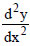= 0exp(-β-γx) = 1, from which x = -β/γ, y = α/2,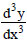= 01- 4 exp(-β-γx) + (exp(-β-γx))2 = 0, from which x1 = -[ln(2+3) + β]/γ, y1 = α(3-3)/6 x2 = -[ln(2-3) + β]/γ, y2 = α(3+3)/6= 0-1+11exp(-β-γx)-11(exp(-β-γx))2+(exp(-β-γx))3 = 0, from which x1 = -[ln(5+26) + β]/γ, y1 = α(3-6)/6, x2 = -β/γ, y2 = α/2, x3 = -[ln(5-26) + β]/γ, y3 = α(3+6)/6.

Figure 1 represents the functions (1), (2) and (3) for the estimates of the parameters: a == 517.4 , b =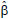= -2.1662, c == 0.1392.

The logistic function considered here (y) is an increasing function within the interval -< x <, without extreme points and with an inflection point (PI). The parameter α is the limit of y when x tends to infinity; y = α is the equation of the upper asymptote. The growth rate function (y') has a maximum point, which abscissa is the abscissa of the inflection point of the curve y, and has two inflection points. The acceleration growth function (y'') presents important information about the growth: at initial x-values we see a great and positive impulse that soon attains a maximum point called hereafter 'maximum acceleration point' (PAM). Then, the acceleration decreases with time x, is null at the inflection point of y and thereafter has negative values; therefore, after the inflection point of y, growth decelerates. The acceleration attains a minimum, called hereafter 'maximum deceleration point' (PDM), and goes toward an inflection point. This point is designated in Figure 1 as PDA = asymptotic deceleration point; after it, the deceleration is very slow and y'' tends to zero as x tends to infinity. For this reason, we expect the increases in y to be very small, and possibly without practical application.

The coordinates of the inflection point of the logistic function are the values obtained when the second derivative is equal to zero; equating to zero the third derivative we obtain the coordinates of the points of maximum acceleration, PAM, and of maximum deceleration, PDM, which are the maximum and minimum of the acceleration function, respectively; when the fourth derivative is equal to zero, we obtain the inflection points of the acceleration function. The last inflection point of the acceleration function is denoted here by PDA. Considering the estimates a, b and c of the parameters α, β and γ, respectively, we have:Determination of the non-significant difference point- DNS

This method takes into account the difference y* = a - ŷ, that is, the estimated difference between the asymptote and the curve y, and verifies the point from which this difference can be considered non-significant using a t-test. This point is called here 'non-significant difference point', denoted as DNS. This method proved to be very rigorous, since the obtained DNS points are highly far from the observational data origin, sometimes out of the measured interval, which characterizes extrapolation.

Let Δ = α - y be the difference between the asymptote α and the logistic function (1) and y* = a - ŷ its estimate. The abscissa x0 of the non-significant difference point, DNS, is the solution for the equationfor T = talpha,df , a t-value at alpha significance level, df = degrees of freedom in s2 = estimated error variance. The null hypothesis is H0: Δ = α - y = 0 and the alternative unilateral hypothesis is H1: α - y > 0. To obtain(y*), the estimated y* variance, we use the formula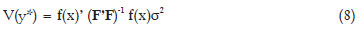where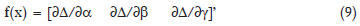that is, the vector of partial derivatives of Δ = α - y relative to the parameters α, β and γ, with 3 × 1 dimension, (F'F)-1σ2 is the variances and co-variances matrix of the parameters estimates of the logistic, with 3 × 3 dimension; σ2 is the error variance. We have

Δ = α - y = αΞ/(1+Ξ), with Ξ = exp(-β-γx)

and the partial derivatives relative to the parametersΔ/α = Ξ/(1+Ξ),Δ/β = -α Ξ/(1+Ξ)2,Δ/γ = -α x Ξ/(1+Ξ)2.

The estimated variances and co-variances matrix of the parameters estimates, a, b and c, iswhere G matrix is obtained from F'F matrix by substituting the parameters for the estimates, and s2 is the residual mean square.

The estimated variance of y* is obtained from (8), (9) and (10), with the estimated parameters in the partial derivatives: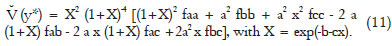Using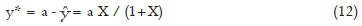we substitute in (7) the equations (11) and (12), for x = x0, obtaining the non-significant difference point, DNS.

Determination of the point using segmented regression - PRS

The segmented regression model is well adequate to estimate growth parameters, according to Robbins (1986) apud Portz et al. (2000). It consists of two parts: an ascending or descending inclined straight line followed by a horizontal line, in which the intersection point determines the break point, called here PRS. For other biological variables, the segmented regression model describes two intersection lines, both with inclination different from zero. The adopted regression will be the one inclination model which is, for n pairs (xi ; yi),

yi = a + b(r-xi) + ei, i=1,2...n1, n1+1, ..., n,

with the restriction (r-xi) = 0 for i > n1+1,

where: n1 = number of observations up to the break point (PRS); a = y-intercept of the horizontal line; -b = angular coefficient of the inclined line; r = abscissa of PRS; ei = experimental error.

Determination of the economic optimum point - PE

Let ŷ = F(x) be the adjusted growth function. The optimum slaughter age of cattle at x = PE, is a solution for the equation

p F'(x) = r p F(x) + k,

where: p = price per kilogram of animal live weight; r = interest rate; k = costs per time unit, per animal.

For the logistic function,where X = exp(-b - cx).

The before-mentioned methods to determine a point sufficiently close to the logistic asymptote will be applied to monthly weight data of heads of cattle of the breeds Flemish, Guernsey and Holstein, obtained from the records of "Seção Técnica de Zootecnia da Escola Superior de Agricultura Luiz de Queiroz / Universidade de São Paulo", Piracicaba, SP. They are summarized in Table 1.

The logistic function adjusted to each example is

yij = α [1 + exp(-β-γxi)]-1 + eij

where yij is the weight (kg) of the animal j in x = age i (months); α, β, γ are parameters and the eij are normal with mean 0 and variance σ2. We will utilize the NLIN procedure of SAS Institute (2009).

This logistic function is largely used in growth studies of animals and plants. Among recent works we cite Paul et al. (2009) with pigs, Oliveira et al. (2000) with Guzerat cattle and Tatar et al. (2009) with goats.

Results and Discussion

Adjustment of the logistic function (1) to data in the examples is in Table 2. The results obtained using the 'proc nlin' of SAS shows the low values of Hougaard skewness coefficients for the parameters. According to Ratkowsky (1989), coefficients lower than 0.1 indicate that the parameter estimator is very close-to-linear in behavior, what is a desirable property for application of parametric tests. Using the estimates of the parameters in Table 2, the mathematical points can be calculated according to (6) (Table 3). The growth at the point of asymptotic deceleration, PDA, is [a(3+6)/6]/a = 90.8 % of the value of the curve asymptote. At this point, therefore, the animal already presents an 'optimum growth'.

Table 4 presents the segmented regression points -PRS - determined by fitting a segmented regression to the data in the examples. The ordinates of segmented regression points are, on average, 89.2 ± 0.85 % of the asymptote value. To determine non-significant difference points, DNS, we use the equation (7)

T =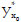/[(y*)],

with the y* variance estimate in (11), for x = x0, and the estimates of the parameters, their variances and co-variances presented in Table 2. Considering the degrees of freedom in error mean squares in Table 2, and 5% significance level, talpha,df values (one-tailed tests) are 1,675 for Example 1 and 1,650 for the others.

The abscissas of non-significant difference points, DNS, for Examples 1 to 4 are all beyond the interval of data observation, which suggests that this method is very rigorous to determine a 'stop point'. This rigor can be reduced if we consider a y* difference between ŷ and a percentage, p, of the estimated asymptote. In this case, we have: y* = pa - ŷ and(y*) = (1+X)-4 [faa Z2 (1+X)2 + fbb a2 X2 + fcc a2 x2X2 - 2 fab a Z X (1+X) - 2 fac a Z x X (1+X) + 2 fbc a2 x X2 ], with Z = p(1+X)-1 and X = exp(-b-cx).

The non-significant difference (DNS) values for p = 0.90 and p = 0.95 are in Table 4. The economic optimum point, PE, was determined using equation (13) with p/k = 0.353 and r = 0.01. We obtain PE = (30.1; 457.2) for Example 1; this value x = 30.1 is very close to the other points in Table 4, for Example 1. Figure 2 plots the critical points for the logistic function adjusted to the data in Example 1. Considering the proportion ŷ/a for each point, on average, for all four examples, we have 88.0 ± 0.28, 89.2 ± 0.85, 90.8, and 93.7 ± 0.21 % for the points non-significant difference, 0.90 (DNS), segmented regression, (PRS), asymptotic deceleration, (PDA) and non-significant difference 0.95 (DNS), respectively (Table 4).

The method used to obtain the non-significant difference (DNS) point yields values extremely close to the asymptote. However, if a proportion of the asymptote, such as 0.90 and 0.95, is considered, the obtained values are close to the others, segmented regression (PRS) and asymptotic deceleration (PDA). The asymptotic deceleration point is highly close to segmented regression point, the latter being determined using a method already known in literature (Table 4 and Figure 2). Nevertheless, the asymptotic deceleration point has the advantage of being a mathematical point with important biological meaning. The point of asymptotic deceleration, together with the inflection point can, therefore, be used in discussions about biological growth curves.

Conclusions

The point of the logistic growth curve corresponding to the beginning of an asymptotic deceleration (PDA) can be used as a criterion to determine when the growth attains a value sufficiently close to the asymptote, so that we can ignore posterior increases. It has the advantage over the other points of presenting biological meaning, besides being an easy-to-calculate point, only the parameters estimates of the function have to be known. The PDA values obtained in the considered examples agree with those obtained using the segmented regression method (PRS). The point of non-significant difference at 5% level between the curve and the estimated asymptote, DNS, is very close to this asymptote. For this reason, it is not a good criterion to determine a 'stop point' in growth; an alternative, in this case, is to employ a proportion of the asymptote, such as 0.90 or 0.95, by instance, which leads to results similar to the remaining points.

Acknowledgements

To "CAPES Pró-Equipamentos nº 1, 01/2007" program, for the equipments provided.

Accepted January 05, 2010

• Chatkin, J.M.; Fiterman, J; Fonseca, N.A.; Fritscher, C.C. 2001. Change in asthma mortality trends in children and adolescents in Rio Grande do Sul: 1970-1998. Jornal de Pneumologia 27: 89-93. (in Portuguese, with abstract in English).
• Gregorczyk, A. 1998. Richards plant growth model. Journal of Agronomy and Crop Science 181: 243-247.
• Oliveira, H.N.; Lôbo, R.B.; Pereira, C.S. 2000. Comparison of non-linear models for describing growth of Guzerat beef cattle females. Pesquisa Agropecuária Brasileira 35: 1843-1851. (in Portuguese, with abstract in English).
• Paul, A.K.; Kundu, M.G.; Singh, S.; Singh, P. 2009. Heritability of growth curve parameters of pigs. Indian Journal of Animal Sciences 79: 716-719.
• Portz, L.; Dias, C.T.S.; Cyrino, J.E.P. 2000. A broken-line model to fit fish nutrition requirements. Scientia Agricola 57: 601-607.
• Ratkowsky, D.A. 1989. Handbook of Nonlinear Regression Models. Marcel Dekker, New York, NY, USA.
• SAS Institute. The SAS System: Release 9.1. SAS Institute, Cary, NC, USA.
• Tatar, A.M.; Dellal, G.; Baritci, I.; Ozkan, M.; Tekel, N. 2009. Journal of Animal and Veterinary Advances 8: 213-216.
• *
Corresponding author <

# Publication Dates

• Publication in this collection
13 Jan 2011
• Date of issue
Feb 2011

• Accepted
05 Jan 2010